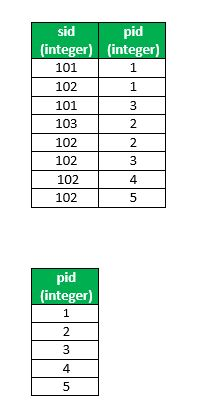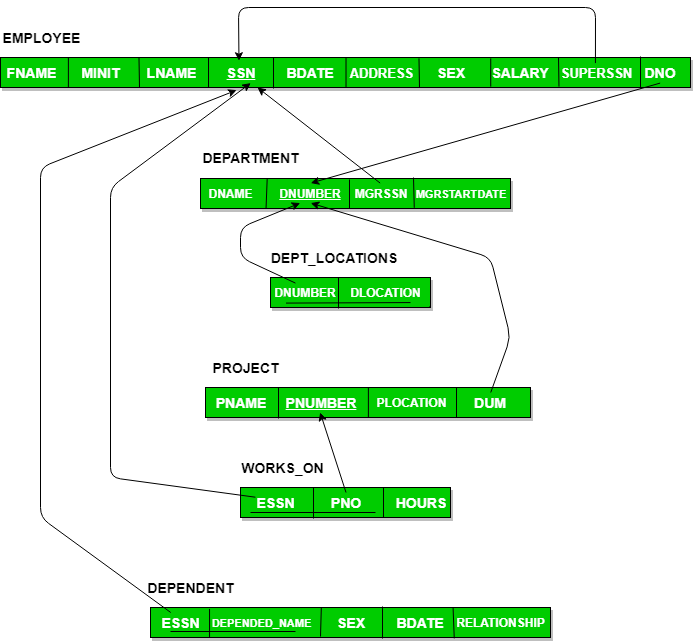GFG App
Open AppBrowser
Continue

# SQL | DIVISION

Division is typically required when you want to find out entities that are interacting with all entities of a set of different type entities.
The division operator is used when we have to evaluate queries which contain the keyword ‘all’.

Some instances where division operator is used are:

• Which person has account in all the banks of a particular city?
• Which students have taken all the courses required to graduate?

In all these queries, the description after the keyword ‘all’ defines a set which contains some elements and the final result contains those units who satisfy these requirements.

Important: Division is not supported by SQL implementations. However, it can be represented using other operations.(like cross join, Except, In )

SQL Implementation of Division

Given two relations(tables): R(x,y) , S(y).
R and S : tables
x and y : column of R
y : column of S

R(x,y) div S(y) means gives all distinct values of x from R that are associated with all values of y in S.
Computation of Division : R(x,y) div S(y)
Steps:

• Find out all possible combinations of S(y) with R(x) by computing R(x) x(cross join) S(y), say r1
• Subtract actual R(x,y) from r1, say r2
• x in r2 are those that are not associated with every value in S(y); therefore R(x)-r2(x) gives us x
that are associated with all values in S

Queries

1. Implementation 1:
```SELECT * FROM R
WHERE x not in ( SELECT x FROM (
(SELECT x , y FROM (select y from S ) as p cross join
(select distinct x from R) as sp)
EXCEPT
(SELECT x , y FROM R) ) AS r );

```
2. Implementation 2 : Using correlated subquery
```SELECT * FROM R as sx
WHERE NOT EXISTS (
(SELECT p.y FROM S as p )
EXCEPT
(SELECT sp.y FROM  R as sp WHERE sp.x = sx.x ) );

```

Relational algebra

```Using steps which is mention above:
All possible combinations
r1 ← πx(R) x S
x values with “incomplete combinations”,
r2x ← πx(r1-R)
and
result ← πx(R)-r2x

R div S = πx(R)- πx((πx(R) x S) – R)
```

### Examples

Supply SchemaHere sid means supplierID and pid means partsID.
Tables: suppliers(sid,pid) , parts(pid)

1. Find suppliers that supply all parts.

Ans 1 : Using implementation 1

```SELECT * FROM suppliers
WHERE sid not in ( SELECT sid FROM ( (SELECT sid, pid FROM (select pid from parts) as p
cross join
(select distinct sid from supplies) as sp)
EXCEPT
(SELECT sid, pid FROM supplies)) AS r ); ```

Ans 2: Using implementation 2

```SELECT * FROM suppliers as s
WHERE NOT EXISTS (( SELECT p.pid FROM parts as p )
EXCEPT
(SELECT sp.pid FROM supplies sp WHERE sp.sid = s.sid ) );```

Company schema2. List employees who work on all projects controlled by dno=4.

Ans 1. Using implementation 1

```SELECT * FROM employee AS e
WHERE ssn NOT IN (
SELECT essn FROM (
(SELECT essn, pno FROM (select pno from project where dno=4)
as p cross join (select distinct essn from works_on) as w)
EXCEPT (SELECT essn, pno FROM works_on)) AS r ); ```

Ans 2. Using implementation 2

```SELECT * FROM employee AS e
WHERE NOT EXISTS (
(SELECT pno FROM project WHERE dno = 4)
EXCEPT
(SELECT pno FROM works_on WHERE essn = e.ssn) );```

Important :
For division correlated query seems simpler to write but may expensive to execute.

Some more Examples.

1. List supplier who supply all ‘Red’ Parts.(supply schema)
2. Retrieve the names of employees, who work on all the projects that ‘John Smith’ works (company schema)